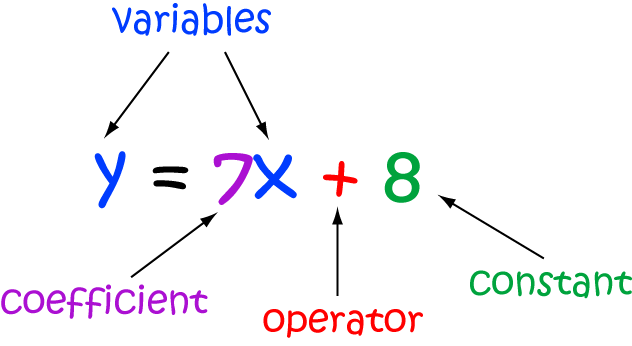# Definition of OperatorAn operator is a mathematical symbol that tells you what operation to perform on the variables that it is applied to.

In the equation $y = 7x + 8$, the operator is +, so the operation to be performed is addition.

### Description

The aim of this dictionary is to provide definitions to common mathematical terms. Students learn a new math skill every week at school, sometimes just before they start a new skill, if they want to look at what a specific term means, this is where this dictionary will become handy and a go-to guide for a student.

### Audience

Year 1 to Year 12 students

### Learning Objectives

Learn common math terms starting with letter O

Author: Subject Coach
You must be logged in as Student to ask a Question.